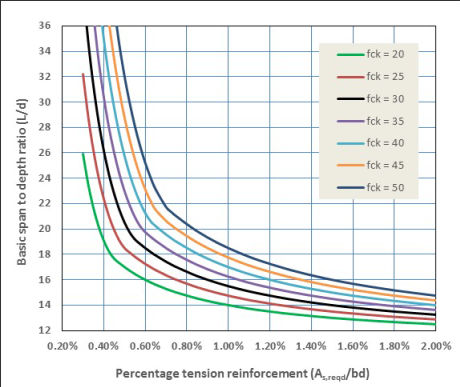# Design aids for determining deflection

Eurocode 2 has two alternative methods for checking deflection. Either:

• a limiting span-to-depth ratio may be used or
• the theoretical deflection can be assessed using the expressions given in the Code

Here the span-to-effective-depth (L/d) method is explained. For the ‘rigorous’ method see How to Design Concrete Structures using Eurocode 2: 8 Deflection calculations.

The span-to-effective-depth (L/d) method

In simple terms, the current EN1992 L/d method means verifying that:

 Allowable L/d = N x K x F1 x F2 x F3 ≥ actual L/d

where

 N = basic span-to-effective-depth ratio derived for K = 1.0 and p'. from the formulae : if ρ ≤ ρ0 N = L/d = K[11 + 1.5fck0.5 ρ 0/ ρ + 3.2 fck0.5(ρ 0/ ρ – 1)1.5] or if ρ > ρ0 N = L/d = K[11 + 1.5 fck 0.5 ρ0/( ρ – ρ') + fck0.5(ρ'/ ρ0)0.5/12] For ρ’ =0, N may be determined from Figure 1 where L = span d = effective depth ρ0 = fck0.5/1000 ρ  = As,req/bd ρ’ = As2/bd K = factor to account for structural system. See Table 1 F1 = factor to account for flanged sections. When beff/bw = 1.0, factor F1 = 1.0. When beff/bw is greater than 3.0, factor F1 = 0.80. For values of beff/bw between 1.0 and 3.0, interpolation may be used. . F2 = factor to account for brittle partitions in association with long spans. Generally F2 = 1.0 but if brittle partitions are liable to be damaged by excessive deflection, F2 should be determined as follows: a) in flat slabs in which the longer span is greater than 8.5 m, F2 = 8.5/leff b) in beams and other slabs with spans in excess of 7.0 m, F2 = 7.0/leff F3 = factor to account for service stress in tensile reinforcement = 310/ss1,3a. It is considered conservative to assume that 310/ss = 500 As,prov/(fykAs,req)  where ss  = tensile stress in reinforcement at mid-span (at support for cantilevers) under design load at SLS. Notes In MC2010 this expression is  given in Expression (7.6-23) as 250/ss = 500 As,prov/(fykAs,req) Factors F1, F2 and F3 have been used here for convenience, they are not symbols used in EN1992-1-1. According to the Notes to Table NA.5 of the UK NA, the ratio, F3, is restricted to ≤ 1.5 where ss  is calculated using the characteristic value of serviceability load. Warnings are given that the values of K may not be appropriate when formwork is struck at an early age. L/d may not exceed 40K.

#### Figure 5: Basic span-to-effective-depth ratios, N, for K = 1, ρ' = 0Note: Extract from How to Design Concrete Structures using Eurocode 2 (page 27, Figure 5)

 Table 1 K factors to be applied to basic ratios of span to effective depth for structural system Element K Simply supported beams or slabs 1.0 End span of continuous beams or slabs 1.3 Interior spans of continuous beams or slabs 1.5 Flat slabs (based on longer span) 1.2 Cantilevers 0.4

The use of L/d method ‘will be adequate for avoiding deflection problems in normal circumstances’